# Prisms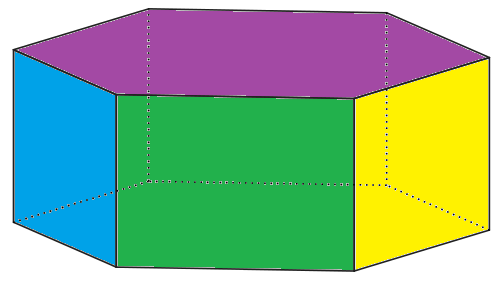Prisms are 3-dimensional (solid) objects with

• 2 bases (ends) that are congruent (identical) and parallel to each other
• All faces flat
• All edges straight
• Cross-sections parallel to the base that are always congruent to the bases.

The bases of a prism are parallel to each other:A cross-section is a view of an object formed by taking a slice through it. In geometry, a cross-section is the shape formed when we cut through a 3-dimensional object with a plane.

For example, the cross-sections of this hexagonal prism, parallel to the base are all hexagonsThe sides of a prism are always parallelograms (4-sided plane shapes with two pairs of parallel sides).

## Right and Oblique Prisms

The sides of a right prism are at right angles to the base. They are always rectangles.

However, it is possible for a prism to have sides that are not at right angles to the base. Then we say that the prism is oblique, and its sides are parallelograms. The bases of an oblique prism are not directly above each other. Oblique prisms lean to the side like the one in this picture.## Regular and Irregular Prisms

Prisms are regular if their bases are regular polygons (flat shapes with straight edges, all of which have the same length).

However, the bases of prisms do not need to be regular polygons. The prism in the picture has its bases shaped like trapeziums. Trapeziums are irregular quadrilaterals, so this prism is irregular.## Examples of Prisms and Their Cross-SectionsA cube is a square prism. The cross-sections of a cube parallel to its bases (pick any face) are squares.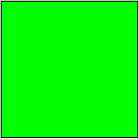Although its bases are trapeziums, this solid is a prism. The cross-sections parallel to its bases are trapeziums.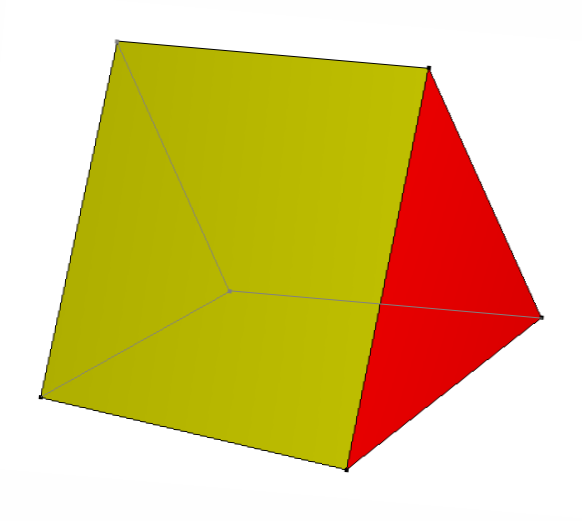Like all prisms, the cross-sections of this triangular prism, parallel to the base, all have the same size and shape as its base. They are all triangles.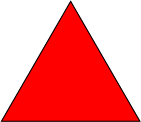## Surface Area of a Prism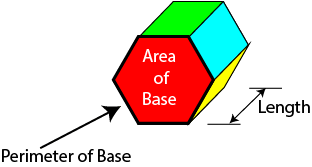The Surface Area of a Prism is given by the following formula:

$\text{Surface Area} = 2 \times \text{Area of Base} + \text{Length} \times \text{Perimeter of Base}$

### Example

Find the surface area of a prism with base area $40 \text{ m}^2$, base perimeter $28 \text{ m}$ and length $5 \text{ m}$.

Solution:

\begin{align*} \text{Surface Area} &= 2 \times \text{Area of Base} + \text{Length} \times \text{Perimeter of Base}\\ &= 2 \times 40 + 5 \times 28\\ &= 136 \text{ m}^2 \end{align*}

## Volume of a PrismThe Volume of a Prism is given by the following formula:

$\text{Volume} = \text{Area of Base} \times \text{Length}$

### Example

Find the volume of a prism with base area $40 \text{ m}^2$ and length $5 \text{ m}$.

Solution:

\begin{align*} \text{Volume} & = \text{Area of Base} \times \text{Length} \\ &= 40 \times 5 \\ &= 200 \text{ m}^3 \end{align*}

### Description

There are several lessons related to 3D geometry such as

1. Euler's formula
2. Vertices, Edges and Faces
3. Volumes of 3D shapes
4. etc

Even though we've titled this lesson series to be more inclined for Year 10 or higher students, however, these lessons can be read and utilized by lower grades students.

### Prerequisites

Understanding of 3D shapes

### Audience

Year 10 or higher, but suitable for Year 8+ students

### Learning Objectives

Get to know 3D Geometry

Author: Subject Coach
You must be logged in as Student to ask a Question.